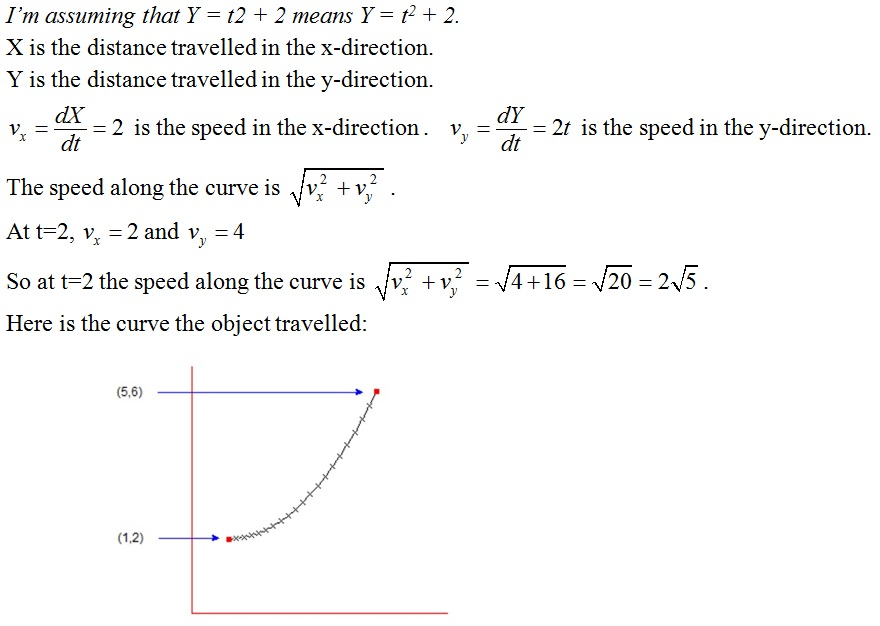# Calculus Problem

Objects moving in two dimensions can be hard to track, unless you use calculus to handle the motion. If an object is moving so its X coordinate follows the equation X = 2t + 1 and its Y coordinate follows the equation Y = t2 + 2, where t is the time in seconds, what is the object’s two-dimensional speed at t = 2 seconds?
How do you solve this problem?Where do you get the formula sqrt((v_x)^2 + (v_y)^2) ?

1 Like

I used Pythagoras’ Theorem.